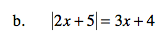Home > A2C > Chapter 11 > Lesson 11.3.1 > Problem11-135

11-135.
1. Solve and check each equation. Homework Help ✎

1.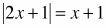2.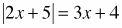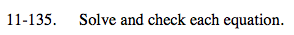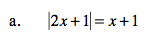Look inside.
2x + 1 = (x + 1) or
2x + 1 = −(x + 1)

x = 0

$x=-\frac{2}{3}$